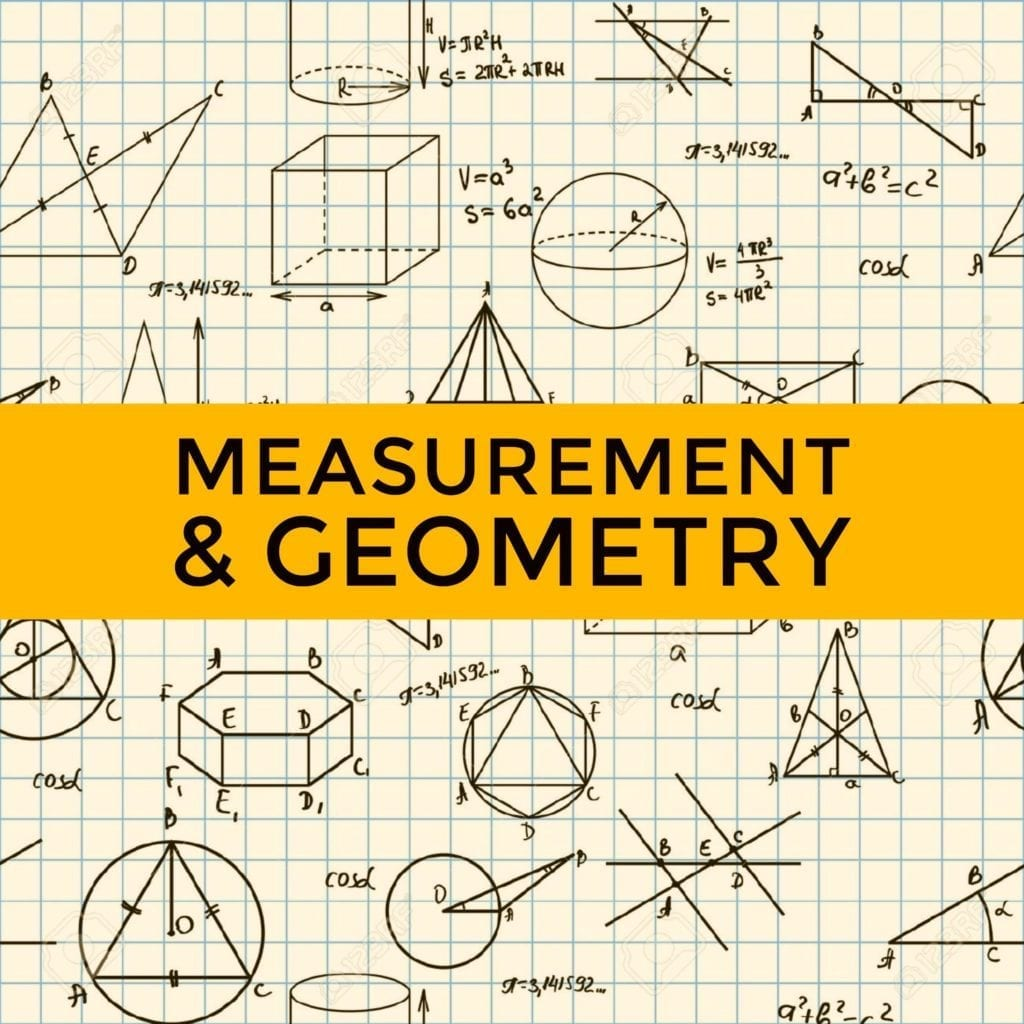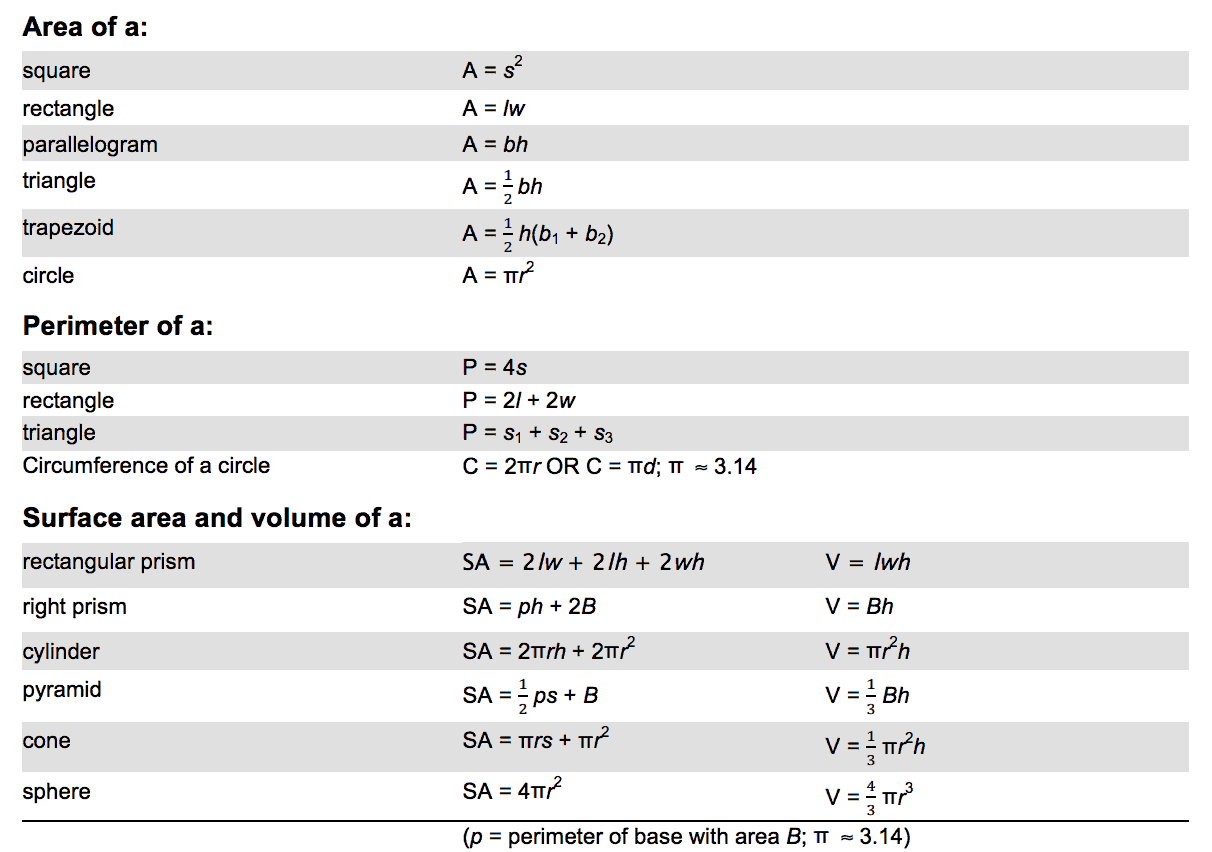# Measurement And Geometry

Measurement and Geometry cover 20-30% of the GED Math Test.

Geometry is a branch of mathematics that deals with the properties of shapes, points, space, positions or angles, and patterns. Studying geometry helps you develop problem-solving skills as well as spatial reasoning, which can be useful in many industries. Measurement and geometry are often used by professionals such as engineers and architects. See our full GED Math study guide here.

In the GED test, you may be given measurements and you will be asked to find the area, perimeter, surface area, or volume of various shapes and figures.

Here are the geometry formulas you need to understand and remember for the GED test:Below are some examples of geometry problems with solutions:

1.  If the circle has a diameter of 10, what is the circumference?

Formula: C = πd

C = 3.14159 (10) *remember that the value of pi is 3.14159.

1. A rectangle is 10 meters long and 5 meters wide. What is its area?

Formula: A = l x w

10 meters x 5 meters

Answer: 50 square meters

1. One side of a square is 7 centimeters. What is its area?

Formula: A = s2

7 cms. x 7 cms.

Answer: 49 square centimeters

To get more tips on how to answer Measurement and Geometry problems on the GED test, watch our GED Math video lessons or take our free GED practice test for math.

Check our Math Blueprint Video Course covering every possible topic for GED Math. It includes +100 videos, +2000 practice questions and loads of information.